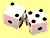Now suppose you want to make Monte Carlo Simulation of unfair coin. You determine specific probability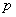to get one of the faces of the coin. If the probability to get zero is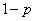and the probability to get one is, we can generate a biased coin using the following formula

Formula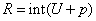Random number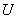that unformly distributed is generated according to previous page . To put this formula in action, I have made a worksheet in MS Excel. You may download it here . The explanation of how to use the worksheet is given below .

### How does the formula work?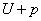maps the value offrom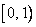into range of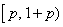. The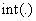function will round down all values below 1 into 0 and round down all values between 1 and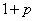into 1.

If the value of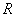is either -1 or 1, then the formula change into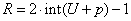.

In general, if we would like to get the value ofis either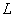or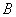, where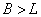with some biased probabilityto get, then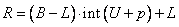The expected mean is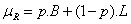and the expected variance is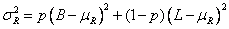or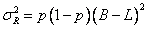### How to use the worksheet?

The sample worksheet uses the two methods above to compute unfair coins. Of course, in many cases you may need to use this more general formula to make 2 integers (not only zero and one, but any numbersand)

Every time you press F9, it generates one sampling experiment of Monte Carlo Simulation. If you delete the word "STOP", every time you press F9, you are not only making one sample of numerical simulation; you will add the statistics of the sampling to all samples results. In this way, actually obtain full simple program of Monte Carlo Simulation.

To use the worksheet fully, delete the word "STOP" and press F9 repeatedly. If you don't delete the STOP, the simulation will still work but the result of all experiments are simply copies of the current experiment. Write any letter or number on that Blue cell to reset the counter.

The formula for mean and variance are recursive. You may see more explanation about this recursive formula in my tutorial on Recursive Statistics . If you are still confuse on how to use iteration in MS Excel, please read my tutorial on MS Excel Iteration .

Preferable reference for this tutorial is

Teknomo, Kardi. Monte Carlo Simulation Tutorial. https:\\people.revoledu.com\kardi\ tutorial\Simulation